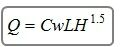Overtopping will begin when the headwater, (Hw) rises to the elevation of the roadway. The overtopping will usually occur at the low point of a sag vertical curve on the roadway. The flow will be similar to flow over a broad crested weir.

It is a simple matter to calculate the flow across the roadway for a given upstream water surface elevation using the weir equation. The problem is that the roadway overflow plus the culvert flow must equal the total flow. A trial and error process is necessary to determine the amount of the total flow passing through the culvert and the amount flowing across the roadway at the same energy grade line.

Culvert Studio uses the following broad crested weir equation:Where:

Q = overtopping flow in cfs (cms)
Cw = broad crested weir coefficient = 3.09 (1.70)
L = crest width in ft (m)
H = Hw – Top Elevation in ft (m)

Where there is a user-defined roadway, the software uses an average depth (H), which is computed as total flow area divided by the top width of flow.